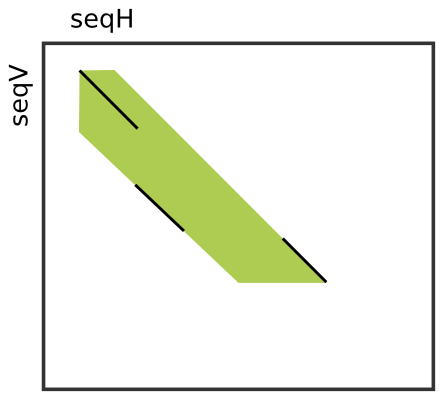Seeds¶

Learning Objective
In this tutorial, you will learn about the seeds-related SeqAn class.
Difficulty
Basic
Duration
15 min
Prerequisites
Sequences

Many efficient heuristics to find high scoring, but inexact, local alignments between two sequences start with small exact (or at least highly similar) segments, so called seeds and extend or combine them to get larger highly similar regions. Probably the most prominent tool of this kind is BLAST [AGM+90], but there are many other examples like FASTA [Pea90] or LAGAN [BDC+03]. You will learn seed-and-extend and many applications including local and global chianing in Seed Extension.

SeqAn’s header file for all data structures and functions related to two-dimensional seeds is <seqan/seeds.h>.

The Seed Class¶The Seed class allows to store seeds. Seeds have a begin and end position in each sequence. Often, two or more close seeds are combined into a larger seed, possibly causing a shift in horizontal or vertical direction between the begin position of the upper left seed and the end position of the lower right seed. For this reason, the Seed class also stores an upper and a lower diagonal to reflect the expansion between those shifted seeds.

The image to the right shows an example where three smaller seeds (black diagonals) were combined (or “chained locally”) into one larger seed (green area).

The Simple Seed specialization only stores the begin and end positions of the seed (left-uppermost and right-lowermost corners of green surface) in both sequences and the upper and lower diagonal. The initial diagonals are not stored. The ChainedSeed specialization additionally stores these information. In most cases, the Simple Seed class is sufficient since the best alignment around the seeds has to be determined using a banded alignment algorithm of the seed infixes anyway.

You can get/set the begin and end position in the horizontal/vertical sequences using the functions beginPositionH, beginPositionV, setBeginPositionH, and setBeginPositionV. The band information can be queried and updated using lowerDiagonal, upperDiagonal, setLowerDiagonal, and setUpperDiagonal. Note, we use the capital letters ‘H’ and ‘V’ to indicate horizontal direction and vertical direction, respectively, while the subject sequence is always considered as the horizontal sequence and the query as the vertical sequence in the context of sequence alignments.

The following program gives an example of creating seeds as well as setting and reading properties.

// Default-construct seed.
Seed<Simple> seed1;
std::cout << "seed1\n"
<< "beginPositionH == " << beginPositionH(seed1) << "\n"
<< "endPositionH == " << endPositionH(seed1) << "\n"
<< "beginPositionV == " << beginPositionV(seed1) << "\n"
<< "endPositionV == " << endPositionV(seed1) << "\n"
<< "lowerDiagonal == " << lowerDiagonal(seed1) << "\n"
<< "upperDiagonal == " << upperDiagonal(seed1) << "\n\n";

// Construct seed with begin and end position in both sequences.
Seed<Simple> seed2(3, 10, 7, 14);
setUpperDiagonal(seed2, -7);
setLowerDiagonal(seed2, -9);
std::cout << "seed2\n"
<< "beginPositionH == " << beginPositionH(seed2) << "\n"
<< "endPositionH == " << endPositionH(seed2) << "\n"
<< "beginPositionV == " << beginPositionV(seed2) << "\n"
<< "endPositionV == " << endPositionV(seed2) << "\n"
<< "lowerDiagonal == " << lowerDiagonal(seed2) << "\n"
<< "upperDiagonal == " << upperDiagonal(seed2) << "\n\n";

The output to the console is as follows.

seed1
beginPositionH == 0
endPositionH == 0
beginPositionV == 0
endPositionV == 0
lowerDiagonal == 0
upperDiagonal == 0

seed2
beginPositionH == 3
endPositionH == 7
beginPositionV == 10
endPositionV == 14
lowerDiagonal == -9
upperDiagonal == -7

Assignment 1¶

Type
Review
Objective
Extend the program above such that seed1 is updated from seed2 and all members (begin positions, end positions, diagonals) are equal to the corresponding member of seed times two. For example, the lower diagonal of seed2 should be 2 * lowerDiagonal(seed1).
Solution
#include <seqan/stream.h>
#include <seqan/seeds.h>
#include <seqan/sequence.h>

using namespace seqan;

int main()
{
// Default-construct seed.
Seed<Simple> seed1;

// Construct seed with begin and end position in both sequences.
Seed<Simple> seed2(3, 10, 7, 14);
setUpperDiagonal(seed2, -7);
setLowerDiagonal(seed2, -9);

// Update seed1.
setBeginPositionH(seed1, 2 * beginPositionH(seed2));
setEndPositionH(seed1, 2 * endPositionH(seed2));
setBeginPositionV(seed1, 2 * beginPositionV(seed2));
setEndPositionV(seed1, 2 * endPositionV(seed2));
setLowerDiagonal(seed1, 2 * lowerDiagonal(seed2));
setUpperDiagonal(seed1, 2 * upperDiagonal(seed2));

// Print resulting seed1.
std::cout << "seed1\n"
<< "beginPositionH == " << beginPositionH(seed1) << "\n"
<< "endPositionH == " << endPositionH(seed1) << "\n"
<< "beginPositionV == " << beginPositionV(seed1) << "\n"
<< "endPositionV == " << endPositionV(seed1) << "\n"
<< "lowerDiagonal == " << lowerDiagonal(seed1) << "\n"
<< "upperDiagonal == " << upperDiagonal(seed1) << "\n\n";

return 0;
}